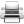# Computational & Multiscale Mechanics of Materials

## Fracture Mechanics Online Class

Other online classes : Aircraft structures

# Numerical Methods

This chapter introduces some existing numerical methods dedicated to the simulation of crack propagation:

• It mainly focuses on Linear Fracture Elastic Mechanics (LFEM) and considers the Finite Element Method (FEM), Cohesive Zone Methods (CZM), and the eXtended Finite Element Method (XFEM);
• The key ideas of Continuum Damage Models (CDM) for ductile materials are also given;
• Finally, multiscale methods are briefly introduced.

# Numerical Methods > Reminder of LEFM

## Definition of elastic fracture

Up to now we have mainly considered elastic fracture mechanics. Strictly speaking, elastic fracture means that the only changes at the material level during the failure are atomic separations. As this way of thinking is too restrictive for real life applications, a pragmatic definition should be: The process zone, which is the region where the inelastic deformations happen, is a small region compared to the specimen sizes (including crack size), and is located at the crack tip. The inelastic deformations may include, among others, plastic flow, micro-fractures, or void growth.

## Summary of the previous lectures

### The Stress Intensity Factor

For linear elastic materials, the linear elastic stress analysis and the asymptotic solution therefore describe the fracture process with accuracy. In particular for the three modes of fracture (I for opening, II for in-plane sliding and III for out-of-plane shearing) the asymptotic solution is expressed in terms of the SIFs following

\begin{equation}\begin{cases} \mathbf{\sigma}^\text{mode i} = \frac{K_i}{\sqrt{2\pi r}} \mathbf{f}^\text{mode i}(\theta) \\ \mathbf{u}^\text{mode i} = K_i\sqrt{\frac{r}{2\pi}} \mathbf{g}^\text{mode i}(\theta) \end{cases},\label{eq:fandg}\end{equation}

### The crack closure integral

The crack closure integral represents the energy required to close the crack on an infinitesimal length $da$.

• If an internal (material) potential exists (linear materials or not), then the energy release rate reads
\begin{equation} G = -\partial_A \left(E_{\text{int}} - W_{\text{ext}}\right) = -\partial_A \Pi_T, \label{eq:GfromPiT}\end{equation}
where $A$ is the crack surface, and where the crack closure integral reads
\begin{equation} \Delta \Pi_T = \int_{\Delta A} \int_{[\![\mathbf{u}]\!]}^{[\![\mathbf{u}+\Delta \mathbf{u}]\!]} \mathbf{t}([\![\mathbf{u}]\!]) \cdot [\![d\mathbf{u}]\!] d(\Delta A) .\label{eq:PiT}\end{equation}
In this expression $\mathbf{t}$ is the traction between the forming crack lips and $[\![d\mathbf{u}]\!]$ is the opening between the crack lips.
• In linear elasticity (thus an internal potential always exists), (\ref{eq:GfromPiT}) simplifies into
\begin{equation} G = -\lim_{\Delta A \rightarrow 0} \frac{1}{2 \Delta A} \int_{\Delta A} \mathbf{t} \cdot [\![\Delta \mathbf{u}]\!] d \partial A \label{eq:GfromPiTLinear},\end{equation}
where $\mathbf{t}$ is the surface traction in the material before the crack propagates, and where $[\![\Delta \mathbf{u}]\!]$ is the opening between the crack lips after the crack has propagated.
• In linear elasticity (thus an internal potential always exists) and if the crack grows straight ahead we have a relation between the energy release rate and the SIFs
\begin{equation} G = \frac{K_I^2}{E'} + \frac{K_{II}^2}{E'} + \frac{K_{III}^2}{2\mu}.\label{eq:GfromSIF} \end{equation}

### The J-integral concept

• The J-integral is the energy that flows towards the crack tip
\begin{equation} J = \int_\Gamma (U(\varepsilon) \mathbf{n}_x - \partial_x \mathbf{u} \cdot \mathbf{T}) dl .\end{equation}
• If an internal potential exists
• The J-integral is path independent if the contour $\Gamma$ embeds a straight crack tip. However, it does not assume on a subsequent growth direction;
• If the crack grows straight ahead then $G = J$;
• In linear elasticity (no assumption on the crack growth direction)
\begin{equation} J = \frac{K_I^2}{E'} + \frac{K_{II}^2}{E'} + \frac{K_{III}^2}{2\mu}.\label{eq:JfromSIF} \end{equation}
• This concept can be extended to plasticity if there is no unloading, as in this case we can define an internal potential (see later).

### Crack Growth

As previously detailed, the growth can be analyzed as follows:
• Crack growth criterion: A crack will grow when the energy release rate is greater than the critical rate of the material. This condition can be written as: \begin{equation} G \geq G_c. \end{equation} In this relation G depends on the sample geometry (including crack length) and boundary conditions, while G C depends on the material:
• Stability of crack growth: A crack is stable if, during the crack propagation, the resistance rate growth is larger than the energy release rate growth: \begin{equation} \partial_a G \leq \partial_a R_c, \end{equation} and unstable otherwise.
• Crack growth direction: The crack growth direction for mixed loadings can be determined by the maximum hoop stress method:
• The crack propagation criterion reads: \begin{equation} \left( \sqrt{2\pi r} \sigma_{\theta\theta}(r,\theta^*) \right) \geq K_c, \end{equation} with $\left.\partial_\theta \sigma_{\theta\theta}\right|_{\theta^*} = 0$ & $\left.\partial_\theta^2 \sigma_{\theta\theta}\right|_{\theta^*} = 0$ ;
• The mixed loading coefficient is defined aunder the form: $\cot \beta^* = \dfrac{K_{II}}{K_{I}}$;
• The maximum hoop stress criterion thus becomes: \begin{equation} \begin{cases} K_C = \left. \sqrt{2\pi r} \sigma_{\theta\theta}(r,\theta^*) \right|_{\theta^*} = K_I \cos^3\dfrac{\theta^*}{2} - \dfrac{3K_{II}}{2} \sin \theta^* cos\dfrac{\theta^*}{2}, \\ 2 \tan \dfrac{\theta^*}{2} - \cot \dfrac{\theta^*}{2} = \tan \beta^* = \dfrac{K_I}{K_{II}} .\end{cases}\end{equation}Last update: September 02, 2015